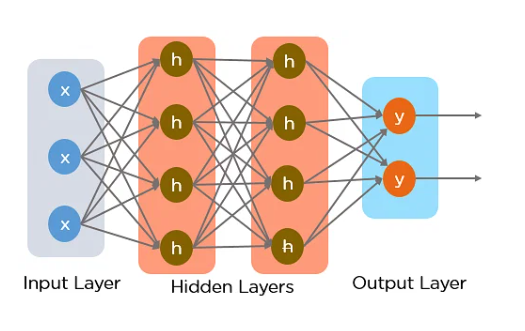# Cardano(ADA) Price Prediction Model- 1 using LSTM

In this article, we will look briefly into a Price prediction Model curated using LSTM(Long Short-Term Memory) algorithm. LSTM is a type of recurrent neural network architecture. It is particularly designed for data involving long-term dependencies. Since we are modeling a price prediction system, we are dealing with time series data which in this case is the historical price data of Cardano’s ADA price. LSTM is known for its efficiency in capturing time series data well. Let us understand how an LSTM algorithm work and why we used it to make this model.

To understand why LSTM was curated, let us first understand how RNNs, in general, work. Recurrent Neural Networks form several connections between nodes(individual processing units) that form a directed cycle. The problem which was faced in the case of using traditional RNNs for data with long-term dependencies was vanishing gradients. During training these RNN models for long-term dependencies, the gradients used to update the weights tend to vanish. LSTM was curated to address this problem by using a more complex network. In an LSTM model, each node works like a memory cell; these nodes are organized into layers for better learning. In Fig. 1, you can see how an LSTM works. An LSTM architecture consists of three gates: input gate, forget gate and output gate.

These gates control the flow of information into, out of, and within the nodes.

1. Input Gate: Depicted by ‘x’ nodes in Fg. 1, determines how much of the input provided to the algorithm should be stored in the memory cell/node.
2. Forget Gate: Decides which information received from the previous memory cell state should be forgotten.
3. Output Gate: It determines how much of the memory cell state should be output as the current prediction.Fig. 1 (Source: https://www.simplilearn.com/tutorials/deep-learning-tutorial/rnn)

From Fig. 1, you can notice that the architecture consists of 3 types of layers, namely: The input layer, Hidden layers, and the output layer.

1. Input Layer: This layer is responsible for receiving the initial input data. Each input layer can contain multiple nodes; each node in this layer corresponds to a specific feature in the input.
2. Hidden Layers: One LSTM model can contain one or more hidden layers. This is where nodes capture and learn from the data. It is in this layer that most of the computational and memory operations take place.
3. Output Layer: This layer processes the information given out by the hidden layers and produces the final prediction.

Methodology

To train the prediction model, we have used historical price data of the ADA coin. This data is retrieved using Coinranking API for the period 29-06-2018 to 29-06-2023. We have used everyday price data collected at the same timestamp for 5 years. Fig. 2 shows this data as a line graph to understand the fluctuation in the token’s price over the years.

Fig. 2

As we can notice, the price of Cardano(ADA) fluctuated between \$0 to \$3 in these 5 years. After collecting the data, we curated an LSTM model to build a prediction model. The LSTM model was built with a single layer for decreased complexity. The algorithm is taught using 80% of the entire dataset. This subset is called the training dataset.

After training the model, the model is made to predict the token’s price on the 20% of data that was kept hidden from the system. This subset is called the testing dataset. This is done to understand how well the algorithm will predict the price for future dates. As this data was never fed to the system, it works like future data. Fig. 3 is the graph plotted with both the predicted and the actual prices of the token on those dates.

Fig. 3

Here, we can notice that the algorithm has done a fair job of understanding the price pattern, but the predictions are not that close to the actual price of the token. The Mean Square Error for these predictions is calculated at around 0.001357. This is generally the scenario in case of overfitting data. Overfitting happens when the algorithm ‘memorizes’ the patterns ‘too well’ from the training dataset, it fails to modify itself for future patterns, and therefore, the model gives greater error in making predictions for the testing dataset than the training dataset. Ideally, when the error between the predictions made on the training dataset and that made on the testing dataset is minimum, that symbolizes that there is no overfitting or underfitting of data. To clarify if our model is experiencing overfitting, we plotted a graph for predictions made on the training dataset and the actual price data, shown in Fig. 4.

Fig. 4

Clearly, the two lines are falling exactly on each other, signifying that the error, in this case, is way less than in the case of testing data. Therefore, it proves to be a case of Overfitting data.

To solve this issue, we can increase the complexity of the model so that it can learn better. This is done by adding layers to the LSTM model. Now, the algorithm is made using 3 layers instead of 1. Fig. 5 shows the output of Predicted price values given by this new model.

Fig. 5

Here, the MSE is calculated to be 0.0002817, which is less than the previous model. Even by looking at the graph(Fig. 5), we can analyze that this new model with increased layers is doing a good job of predicting the token’s price, as the orange line of prediction is almost tracing the blue line of the actual price data. Therefore, we can conclude that the developed LSTM model makes near-to-actual price predictions for Cardano(ADA). Although the crypto market is volatile in nature and can be affected by numerous reasons, the prediction models should not be solely trusted for trading in the market. Investors should carry out thorough research and analyze the market sentiments before investing in any cryptocurrency.